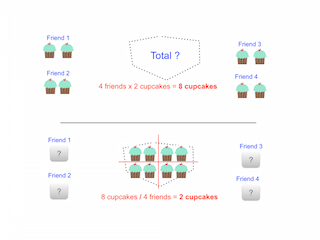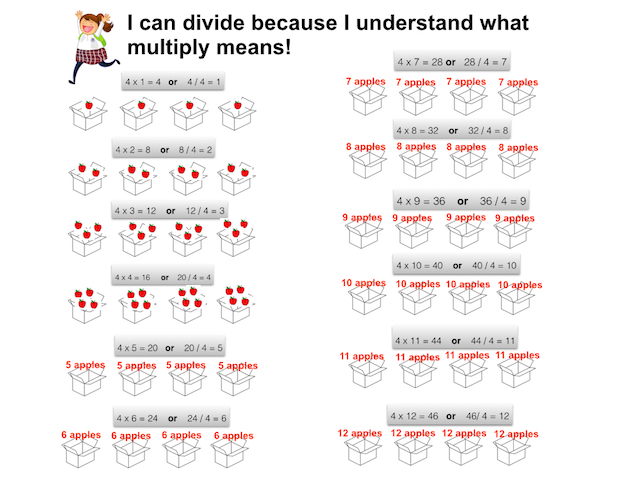# How to Divide by 4

Instructor: Carla Palomino
In this lesson, you'll learn an easy way to divide by 4 and how multiplication and division work together to help you solve division by 4 problems. If you follow the steps in this lesson, you'll be solving division by 4 problems in record time!

## What Does It Mean to Divide by 4?

Every time you hear you have to divide by 4, that means something will be split into 4 equal parts or 4 equal groups. There is a trick you can use to divide by 4: the rule is to divide by 2 twice. For example, if you want to divide 12 by 4, you simply divide 12 by 2, which gives you 6, and then divide that number by 2, which, in this case, gives you 3. Easy!

## Connection Between Division and Multiplication

To get ready to solve any division by 4 problem, it is really important to understand the connection between multiplication and division. They are opposite operations, and both have to do with groups of equal size.

#### Multiplication

If you have 4 friends and you want to buy 2 cupcakes for each of them, you'll need to buy 8 cupcakes. Here, you are multiplying 4 friends by 2 cupcakes, which equals 8 cupcakes: 4 x 2 = 8.

#### Division

The opposite of multiplication is division. You have already bought 8 cupcakes. Now, you want to split them between 4 friends. To do this, you'll have to divide 8 cupcakes by 4 friends, which equals 2 cupcakes for each one: 8 / 4 = 2.## How to Divide by 4

To divide by 4 means finding out how many 4s there are in your total number. In order to fully understand division by 4, it is really important to review what a table of 4 means.Now that we understand what we are doing when we divide by 4, let's practice with a real problem.

Imagine you have 36 apples, and you want to split them evenly into 4 boxes. How many apples will you put in each box?

To solve this problem, keep the following information in mind:

To unlock this lesson you must be a Study.com Member.

### Register to view this lesson

Are you a student or a teacher?

Back

### Resources created by teachers for teachers

Over 30,000 video lessons & teaching resources‐all in one place.Video lessonsQuizzes & WorksheetsClassroom IntegrationLesson Plans

I would definitely recommend Study.com to my colleagues. It’s like a teacher waved a magic wand and did the work for me. I feel like it’s a lifeline.

Jennifer B.
TeacherCreate an account to start this course today
Used by over 30 million students worldwide